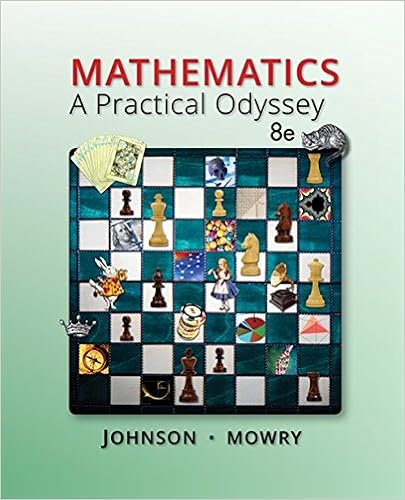# Collect the sample data and compute the value of the

• Notes
• 60
• 100% (1) 1 out of 1 people found this document helpful

This preview shows page 51 - 58 out of 60 pages.

##### We have textbook solutions for you!
The document you are viewing contains questions related to this textbook.The document you are viewing contains questions related to this textbook.
Chapter 1 / Exercise 5
Mathematics: A Practical Odyssey
Johnson/MowryExpert Verified
Collect the sample data and compute the value of the test statistic In the Valentine Day case, the value of the test statistic was calculated to be z = 1.00 5. Calculate the p-value by corresponding to the test statistic value In the Valentine Day case, the area under the standard normal curve in the left-hand tail to the left of the test statistic value z = 1.00 The area is 0.1587 The p-value is 0.1587 · 2 = 0.3174 LO9-3
##### We have textbook solutions for you!
The document you are viewing contains questions related to this textbook.The document you are viewing contains questions related to this textbook.
Chapter 1 / Exercise 5
Mathematics: A Practical Odyssey
Johnson/MowryExpert Verified
9-52 Steps Using a p-value to Test a “Not Equal To” Alternative Continued 5. Continued That is, if H 0 is true, probability is 0.3174 of obtaining a sample whose mean is at least as extreme as 326 This probability is not so low as to be evidence that H0 is false and should be rejected 6. Reject H 0 if the p-value is less than a In the Valentine Day case, α was 0.05 Calculated p-value of 0.3174 is greater than α This implies that the test statistic z = 1.00 is greater than the rejection point z 0.025 = 1.96 Therefore do not reject H 0 at the α = 0.05 significance level LO9-3
9-53 9.3 t Tests about a Population Mean: σ Unknown Assume the population being sampled is normally distributed, or sample size n is large (30 or more) The population standard deviation σ is unknown, as is the usual situation If the population standard deviation σ is unknown, then it will have to estimated from the sample standard deviation Under these two conditions, have to use the t distribution to test hypotheses LO9-4: Use critical values and p-values to perform a t test about a population mean when σ is unknown.
9-54 Defining the t Statistic: σ Unknown Let x be the mean of a sample of size n with standard deviation s; calculate the standard error SE = s/√n. μ 0 is the claimed value of the population mean Define a new test statistic If the population being sampled is normal or n is large, and s is used to estimate σ, then … The sampling distribution of the t statistic is a t distribution with n 1 degrees of freedom s n x n s x SE x t ) ( 0 0 0 LO9-4
9-55 t Tests about a Population Mean: σ Unknown Alternative Reject H 0 if: p-value H a : μ > μ 0 t > t Area under t distribution to right of t H a : μ < μ 0 t < t Area under t distribution to left of t H a : μ μ 0 |t| > t /2 * Twice area under t distribution to right of |t| t α , t α /2 , and p-values are based on n 1 degrees of freedom (for a sample of size n ) * either t > t α /2 or t < t α /2 LO9-4
9-56 9.4 z Tests about a Population Proportion Alternative Reject H 0 if: p-value H a : p > p 0 z > z Area under t distribution to right of z H a : p < p 0 z < z Area under t distribution to left of z H a : p p 0 |z| > z /2 * Twice area under t distribution to right of |z| Where the test statistics is * either z > z α /2 or z < z α /2 n p p p p ˆ z 0 0 0 1 LO9-5: Use critical values and p-values to perform a large sample z test about a population proportion.
9-57 9.5 Type II Error Probabilities and Sample Size Determination (Optional) Want the probability β of not rejecting a false null hypothesis Want the probability β of committing a Type II error 1 - β is called the power of the test Assume that the sampled population is normally distributed, or that a large sample is taken Test… H 0 : μ = μ 0 vs H a : μ < μ 0 or H a : μ > μ 0 or H a : µ ≠ µ 0 Want to make the probability of a Type I error equal to α
•••